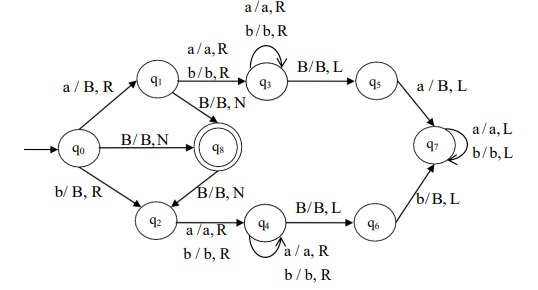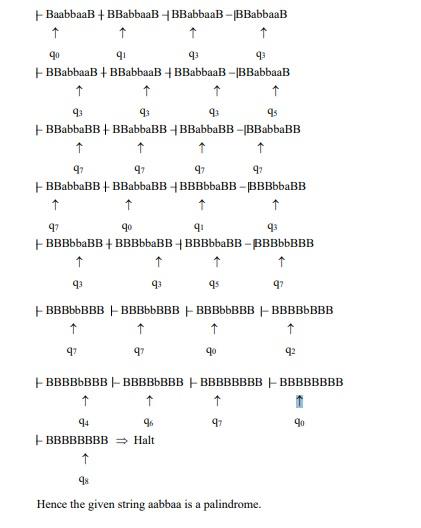# Design a TM which recognizes palindromes over = {a, b}

## Algorithm

Step 1 - If there is no input, reach the final state and halt.

Step 2 - If the input = “a‟, then traverse forward to process the last symbol = “a‟. Convert both a‟s to B‟.

Step 3 - Move left to read the next symbol.

Step 4 - If the input = “b‟, replace it by B and move right to process its equivalent “B‟ at the rightmost end.

Step 5 - Convert the last ’b’ to ‘B’.

Step 6 - Move left and process step 2 – 5 until there are no more inputs to process.

Step 7 - If the machine reaches the final state after processing the entire input string, then the string is a palindrome that halts the machine.

## Turing Machine

The turing machine is as follows −The Turing machine, M is given by M = (Q, Σ, Γ, δ, q0, B, F)

Where,

• Q = {q0, q1, q2, q3, q4, q5, q6, q7, q8}

• Σ = {a, b}

• Γ = {a, b, B}

• δ ⇒ Given by the above mentioned transition diagram,

• q0 = {q0}

• B = {B}

• F = {q8}

Consider a string aabbaa, as shown below −